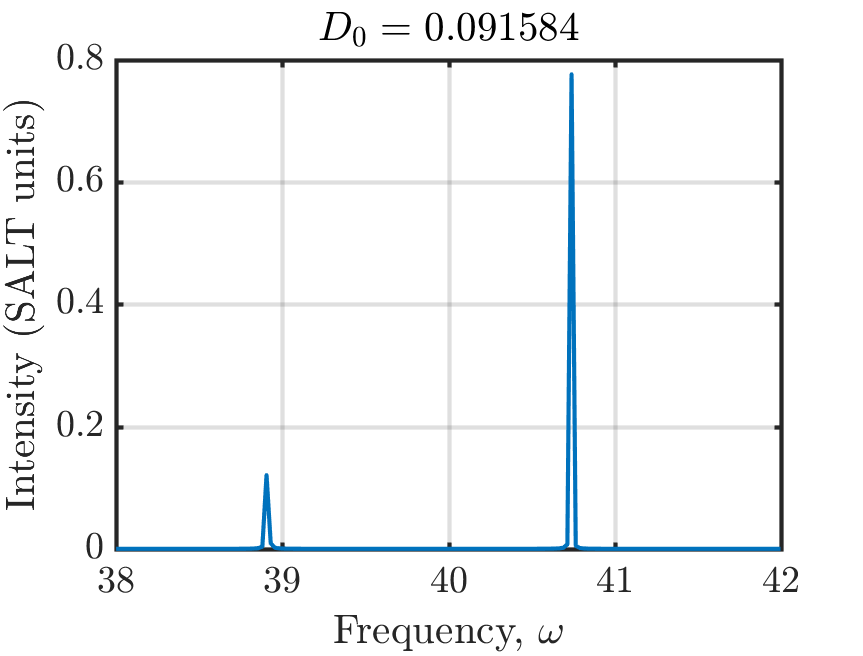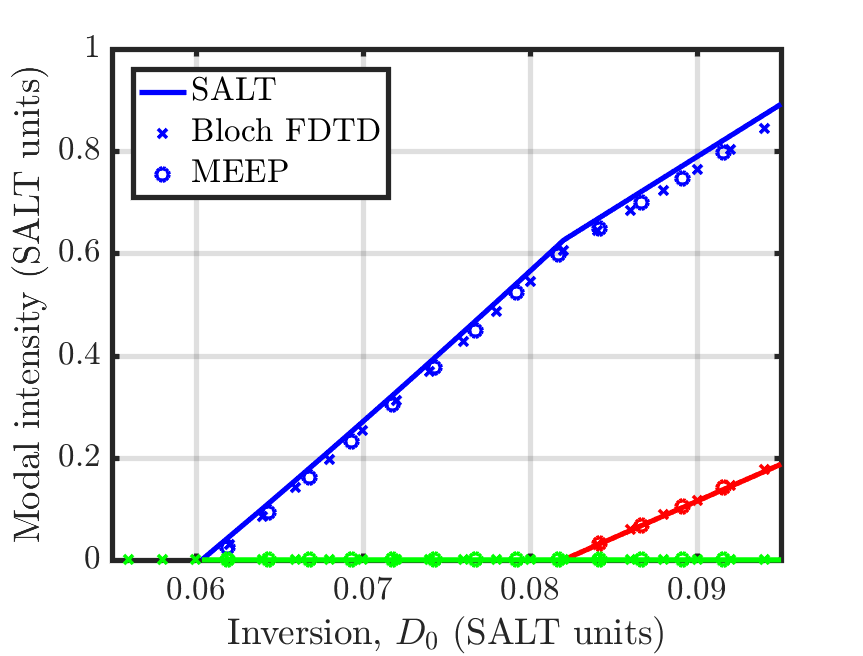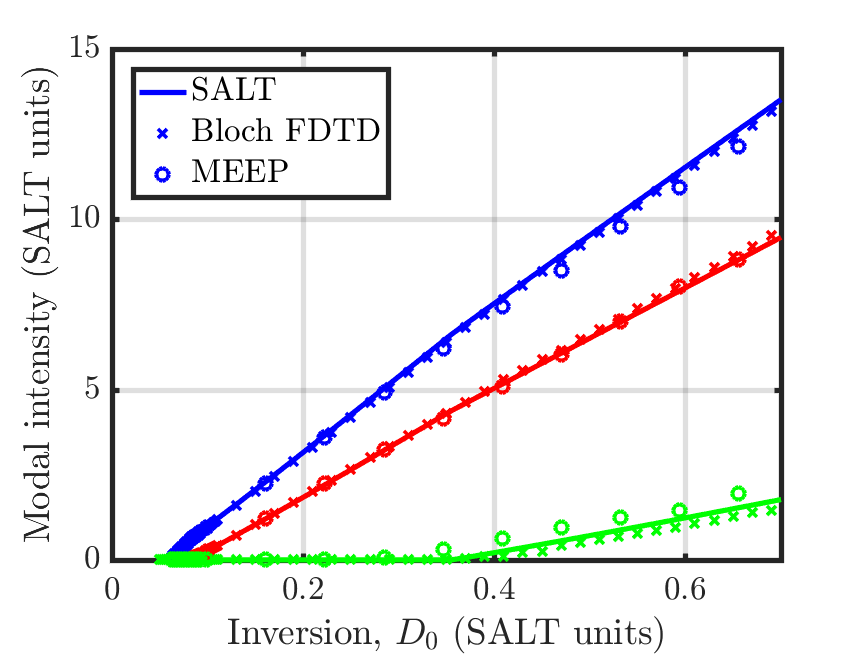# Multilevel-Atomic Susceptibility

This tutorial demonstrates Meep's ability to model saturable gain and absorption via multilevel atomic susceptibility. This is based on a generalization of the Maxwell-Bloch equations which involve the interaction of a quantized system having an arbitrary number of levels with the electromagnetic fields. The theory is described in arXiv:2007.09329 and Materials/Saturable Gain and Absorption. This example involves computing the lasing thresholds of a two-level, multimode cavity in 1d similar to the structure described in Figure 2 of Optics Express, Vol. 20, pp. 474-88, 2012.

First, the cavity consists of a high-index medium ($n = 1.5$) with a perfect-metallic mirror on one end and an abrupt termination in air on the other.

resolution = 400
ncav = 1.5        # cavity refractive index
Lcav = 1          # cavity length
dpml = 1          # PML thickness
sz = Lcav + dpad + dpml
cell_size = mp.Vector3(z=sz)
dimensions = 1
pml_layers = [mp.PML(dpml, side=mp.High)]


The properties of the polarization of the saturable gain are determined by the central transition's frequency ω$_a$ and full-width half-maximum Γ as well as the coupling constant between the polarization and the electric field σ. Both ω$_a$ and Γ are specified in units of 2π$c$/$a$. As this example involves comparing results from Meep with the steady-state ab initio laser theory (SALT), we show explicitly how to convert between the different variable nomenclatures used in each method.

omega_a = 40                             # omega_a in SALT
freq_21 = omega_a/(2*math.pi)            # emission frequency  (units of 2\pi c/a)

gamma_perp = 4                           # HWHM in angular frequency, SALT
gamma_21 = (2*gamma_perp)/(2*math.pi)    # FWHM emission linewidth in sec^-1 (units of 2\pi c/a)

theta = 1                                # theta, the off-diagonal dipole matrix element, in SALT
sigma_21 = 2*theta*theta*omega_a         # dipole coupling strength (hbar = 1)


To understand the need for the high resolution, let us calculate the central wavelength of the lasing transition inside the high-index cavity relative to the cavity length:

The cavity contains roughly 10 wavelengths. This is an unphysically small cavity. Thus, to ensure that the electric field within the cavity is properly resolved, we have chosen roughly 40 pixels per wavelength, yielding a resolution of 400.

Next, we need to specify the non-radiative transition rates of the two-level atomic medium we're using as well as the total number of gain atoms in the system $N_0$. The non-radiative transition rates are specified in units of $c$/$a$.

rate_21 = 0.005        # non-radiative rate  (units of c/a)
N0 = 37                # initial population density of ground state
Rp = 0.0051            # pumping rate of ground to excited state


For a two-level atomic gain medium, the effective inversion that this choice of parameters corresponds to in SALT units can be calculated as:

The term in parenthesis on the right-hand side is the definition of $D_0$ in normal units, and the additional factor of $|\theta|^2 / \hbar \gamma_\perp$ converts to SALT's units.

transitions = [mp.Transition(1, 2, pumping_rate=Rp, frequency=freq_21, gamma=gamma_21,
sigma_diag=mp.Vector3(sigma_21,0,0)),
mp.Transition(2, 1, transition_rate=rate_21)]
ml_atom = mp.MultilevelAtom(sigma=1, transitions=transitions, initial_populations=[N0])
two_level = mp.Medium(index=ncav, E_susceptibilities=[ml_atom])

geometry = [mp.Block(center=mp.Vector3(z=-0.5*sz+0.5*Lcav)),
size=mp.Vector3(mp.inf,mp.inf,Lcav), material=two_level)]


Definition of the two-level medium involves the MultilevelAtom sub-class of the E_susceptibilities material type. Each radiative and non-radiative Transition is specified separately. Note that internally, Meep treats pumping_rate and transition_rate identically, and you can use them interchangeably, but it is important to specify the from_level and to_level parameters correctly, otherwise the results will be undefined. The choice of these parameters requires some care. For example, choosing a pumping rate that lies far beyond the first lasing threshold will yield large inversion, and thus large gain, which is not realistic, as most physical devices will overheat before reaching such a regime. Meep will still produce accurate results in this regime though. Additionally, choosing the total simulation time is especially important when operating near the threshold of a lasing mode, as the fields contain relaxation oscillations and require sufficient time to reach steady state.

Also important is the definition of σ. When invoking the MultilevelAtom sub-class of the E_susceptibilities material type, we need to specify the three components of sigma_diag. In this example, this is mp.Vector3(sigma_21,0,0). Internally, Meep defines σ as the product of sigma of the MultilevelAtom sub-class (1 in this example) with sigma_diag of each Transition. Thus, this saturable gain media will only couple to, and amplify, the E$_x$ component of the electric field.

The field within the cavity is initialized to arbitrary non-zero values and a fictitious source is used to pump the cavity at a fixed rate. The fields are time stepped until reaching steady state. Near the end of the time stepping, we output the electric field outside of the cavity.

sim = mp.Simulation(cell_size=cell_size,
resolution=resolution,
boundary_layers=pml_layers,
geometry=geometry,
dimensions=dimensions)

sim.init_sim()

def field_func(p):
return 1 if p.z==-0.5*sz+0.5*Lcav else 0

sim.fields.initialize_field(mp.Ex, field_func)

endt = 7000

def print_field(sim):
print("field:, {}, {}".format(sim.meep_time(), fp))

sim.run(mp.after_time(endt-250, print_field), until=endt)


The spectra of the field intensity is shown below.There are two lasing modes above threshold in the vicinity of the center transition frequency ω$_a$=40 as we would expect. Remember, when finding the frequency axis that Meep uses a Courant factor of $\Delta t = 0.5 \Delta x$. We have also converted the electric field to SALT units using:

For two-level gain media, $\gamma_\parallel = \gamma_{12} + \gamma_{21}$. We can also verify that the system is not exhibiting relaxation oscillations by directly plotting the electric field as a function of time and looking for very long time-scale oscillations. In the continuum limit, these modes would appear as Dirac delta functions in the spectra. The discretized model, however, produces peaks with finite width. Thus, we need to integrate a fixed number of points around each peak to calculate the correct modal intensity.

By varying $N_0$ or the pumping rate $R_p$, we can change the total gain available in the cavity. This is used to find the laser's modal intensities as a function of the strength of the gain. We can compare the simulated modal intensity with SALT as well as an independent FDTD solver based on the Maxwell-Bloch equations. All three methods produce results with good agreement close to the first lasing threshold.Further increasing the gain continues to yield good agreement.